# An Exploratory Data Analysis (EDA) on Dota 2

Dota 2 is a multiplayer online battle arena video game developed and published by Valve. In each game, players are required to select an in-game character, generally known as the heroes. This dataset records the overall performance of the heroes. The data was extracted manually from dotabuff.com – the official Dota2 statistics provider on 13/10/2019 into an .xlsx file. The data covers 16 attributes for all 117 heroes available at that time. Both the univariate multivariate EDA will be done and visualisation for the analysis are all done using the ggplot.

library(openxlsx)
df<-read.xlsx('D:/Dataset/datdota/dotabuff.xlsx',sheet = 1, startRow = 1, colNames = T)
head(df,3) 
##                 Hero Matches.Played Pick.Rate Win.Rate KDA.Ratio Kills Deaths
## 1            Abaddon      115975606    0.0553   0.5642      2.91  5.75   6.60
## 2          Alchemist      190340443    0.0907   0.4650      2.23  5.89   7.53
## 3 Ancient Apparition      128959122    0.0614   0.5026      2.69  5.54   7.82
##   Assists Match.Duration Gold./.Minute Experience./.Minute
## 1   13.45       1.687500        379.89              483.94
## 2   10.90       1.708333        611.70              540.32
## 3   15.50       1.768056        315.50              381.71
##   Last.Hits./.10.Minutes Denies./.10.Minutes Hero.Damage./.Minute
## 1                  25.46                0.91               328.41
## 2                  52.01                0.84               366.16
## 3                  12.00                0.93               290.71
##   Tower.Damage./.Minute Hero.Healing./.Minute
## 1                 33.77                 45.62
## 2                 57.82                  0.77
## 3                 10.05                  7.42

## Data Cleaning

### Check for Missing Values

Before the analysis is done, the data is checked for missing or empty cell. This can be done using the colsums() function.

colSums(is.na(df) | df=='')
##                   Hero         Matches.Played              Pick.Rate
##                      0                      0                      0
##               Win.Rate              KDA.Ratio                  Kills
##                      0                      0                      0
##                 Deaths                Assists         Match.Duration
##                      0                      0                      0
##          Gold./.Minute    Experience./.Minute Last.Hits./.10.Minutes
##                      0                      0                      0
##    Denies./.10.Minutes   Hero.Damage./.Minute  Tower.Damage./.Minute
##                      0                      0                      0
##  Hero.Healing./.Minute
##                      0

### Renaming column

R auto,atically replaces space with ‘.’. Some columns name may create additional hassle while reading them later. Take ‘Experience / Minutes’ as example, importing the data using R would convert the column as ‘Experience./.Minute’. Renaming column can be done by various method. If the we are planning to rename one or two column, renaming can easily be done by using the names() function.

new_df <- data.frame(index=1:3,oldName=rnorm(3))
head(new_df)
##   index    oldName
## 1     1 -1.3059777
## 2     2  0.1066659
## 3     3  1.3087354
names(new_df) <- c('newName')
head(new_df)
##   index    newName
## 1     1 -1.3059777
## 2     2  0.1066659
## 3     3  1.3087354

To handle larger dataset, this can be done using the stringr. Replacing period is tricky as ‘.’ in R matches any single character. Including ‘.’ as it is would result in blank column name, hence square bracket [] is needed.

library(stringr)
colname_old <- colnames(df)
colname_new <- str_replace_all(colname_old,pattern = '[.]',replacement = '')
colname_new <- str_replace_all(colname_new,pattern = '/',replacement = 'Per')

colnames(df) <- colname_new
head(df,3)
##                 Hero MatchesPlayed PickRate WinRate KDARatio Kills Deaths
## 1            Abaddon     115975606   0.0553  0.5642     2.91  5.75   6.60
## 2          Alchemist     190340443   0.0907  0.4650     2.23  5.89   7.53
## 3 Ancient Apparition     128959122   0.0614  0.5026     2.69  5.54   7.82
##   Assists MatchDuration GoldPerMinute ExperiencePerMinute LastHitsPer10Minutes
## 1   13.45      1.687500        379.89              483.94                25.46
## 2   10.90      1.708333        611.70              540.32                52.01
## 3   15.50      1.768056        315.50              381.71                12.00
##   DeniesPer10Minutes HeroDamagePerMinute TowerDamagePerMinute
## 1               0.91              328.41                33.77
## 2               0.84              366.16                57.82
## 3               0.93              290.71                10.05
##   HeroHealingPerMinute
## 1                45.62
## 2                 0.77
## 3                 7.42

## Univariate EDA

The WinRate column selected for the univariate exploratory data analysis. The summary() function can be used to obtain some of the basic descriptive statistics. Since R do not have a standard built-in function to compute mode, we can work around that by estimating the mode using using density function for the WinRate. Based on the output, we can tell that the gameplay is somewhat balanced, as the mean, median and mode of the win rate are around 50%.

attach(df)
summary(WinRate)
##    Min. 1st Qu.  Median    Mean 3rd Qu.    Max.
##  0.4011  0.4656  0.4934  0.4916  0.5150  0.5709
density_estimate <- density (WinRate)
mode_value<-density_estimate$x[which.max(density_estimate$y)]
mode_value
##  0.5027889

### Skewness and Kurtosis

One ofthe fundamental task in EDA is to characterize the variablilty and location of a dataset. Apart from the standard descriptive statistics, further characterisation of the dataset can be explained via skewness and kurtosis. Skewness is a measure of symmetry, whereas the kurtosis explains if the data is has a light or heavy tails.

The data has a skewness of -0.005, therefore it is negatively skewed, where the peak leans slightly towards the right, and the longer tail towards the left. The kurtosis of the density plot is 2.55 (less than 3), indicating that the plot is platykurtic, with a thinner tail compared to a normal distribution. These can be validated later using the KDE plot.

library(e1071)
wr_skw <- skewness(WinRate)
wr_skw
##  -0.005136054
wr_kur <- kurtosis(WinRate)
wr_kur
##  -0.4520088

### Kernel Density Estimation (KDE)

To create KDE plot, we can use the ggplot2 library. The output shows that the data is unimodal. The rug plot (red lines at the x axis) represents unduplicated win rate values.

library(ggplot2)
ggplot(df, aes(WinRate)) + geom_histogram(aes(y=..density..), colour="gray", fill="white") +
geom_density()+geom_rug(alpha=0.5,color='Red') +
geom_vline(aes(xintercept=mean(WinRate)), color="blue", linetype="dashed", size=1) +
geom_vline(aes(xintercept=median(WinRate)), color="red", linetype="dashed", size=1) +
geom_vline(xintercept = mode_value,color='purple', linetype="twodash", size =1)+
geom_label(aes(mean(WinRate),4,label='mean'),show.legend = F)+
geom_label(aes(median(WinRate),6,label='median'),show.legend = F)+
geom_label(aes(mode_value,8,label='KDE \nmode'),show.legend = F)+
xlim(0.39,0.61)
## stat_bin() using bins = 30. Pick better value with binwidth.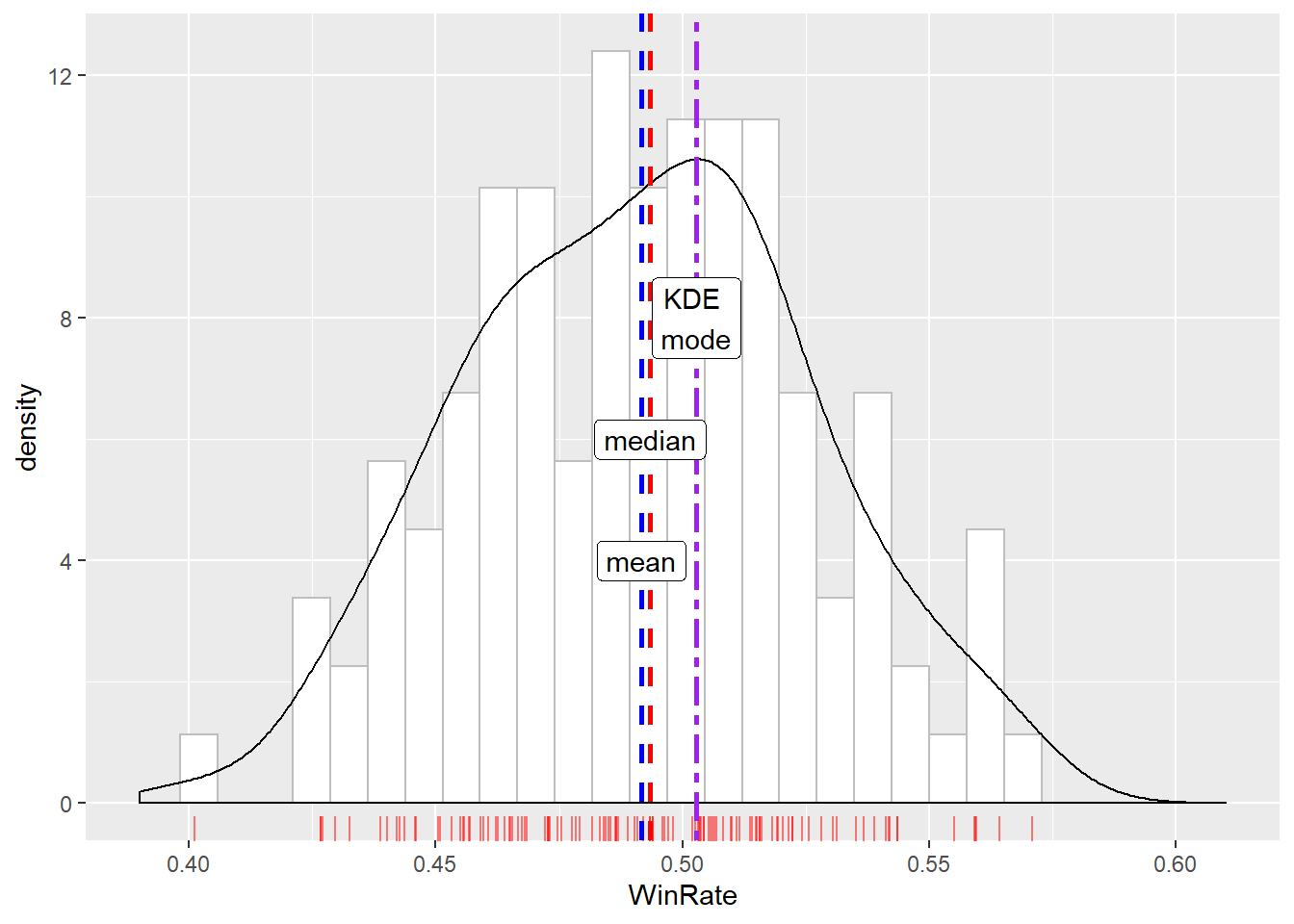### Empirical Cummulative Distribution Function (ECDF)

ECDF shows the distribution of win rate in an ascending order. Based on the plot, only one hero has a win rate below 42% and majority (95%) of the heroes have an average win rate of under 55%.

# ECDF with vline for 25th, 50th, 75th and 95th
# Compute the qth quantile to be displayed on the plot
q25 <- quantile(WinRate, probs=c(.25))
q50 <- quantile(WinRate, probs=c(.5))
q75 <- quantile(WinRate, probs=c(.75))
q95 <- quantile(WinRate, probs=c(.95))

ggplot(df, aes(WinRate))+stat_ecdf(geom='point') + labs(x="WinRate",y="ECDF") +
geom_vline(xintercept=q25,linetype="dashed",colour='red',size =1)+
geom_label(aes(q25,0.1,label='25th Percentile'),show.legend = F)+
geom_vline(xintercept=q50,linetype="dashed",colour='blue',size =1)+
geom_label(aes(q50,0.3,label='50th Percentile'),show.legend = F)+
geom_vline(xintercept=q75,linetype="dashed",colour='green',size =1)+
geom_label(aes(q75,0.55,label='75th Percentile'),show.legend = F)+
geom_vline(xintercept=q95,linetype="dashed",colour='black',size =1)+
geom_label(aes(q95,0.8,label='95th Percentile'),show.legend = F)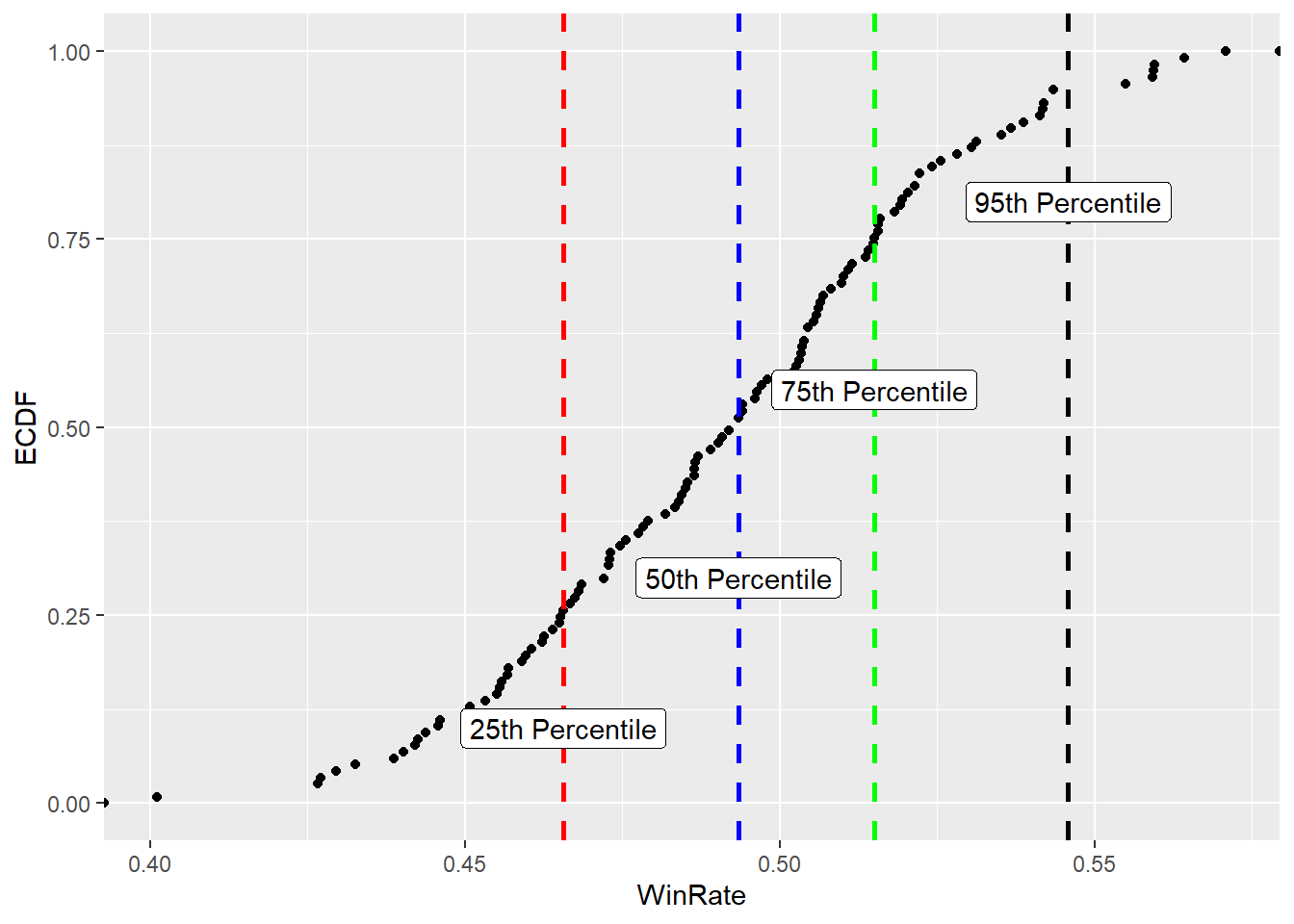## Multivariate EDA

Multivariate EDA examines the relationship between variables. To simplify the visualisation and analysis, only GoldPerMinute (GPM), ExperiencePerMinute (XPM) and KDARatio are selected. Based on the correlation matrix, all the variables shows positive correlation. GPM - XPM pair has the highest correlation, followed by XPM - KDA, and GPM - KDA. The high correlation between GPM - XPM is consistent with the logic of the game, where heroes obtain gold or experience by killing the creeps or opponent heroes.

# select 3 columns KDA.Ratio,Gold./.Minute, Experience./.Minute
# Create a dataframe using the columns selected
multivariate<-data.frame( GoldPerMinute, ExperiencePerMinute,KDARatio)
cor(multivariate[1:3])
##                     GoldPerMinute ExperiencePerMinute  KDARatio
## GoldPerMinute           1.0000000           0.8862935 0.2959903
## ExperiencePerMinute     0.8862935           1.0000000 0.4473213
## KDARatio                0.2959903           0.4473213 1.0000000

To visualise the correlation betwenen the variables, pair plot is created. The lower triangle shows the scatterplot of each pairs and the best fitted regression line, the diagonal summarises each of the variables using kernel density estimation plot, and the upper triangle shows the 2D density diagram.

library(GGally)
## Registered S3 method overwritten by 'GGally':
##   method from
##   +.gg   ggplot2
ggpairs(multivariate,upper=list(continuous = 'density'),
lower = list(continuous='smooth'),
diag = list(continuous ='densityDiag'))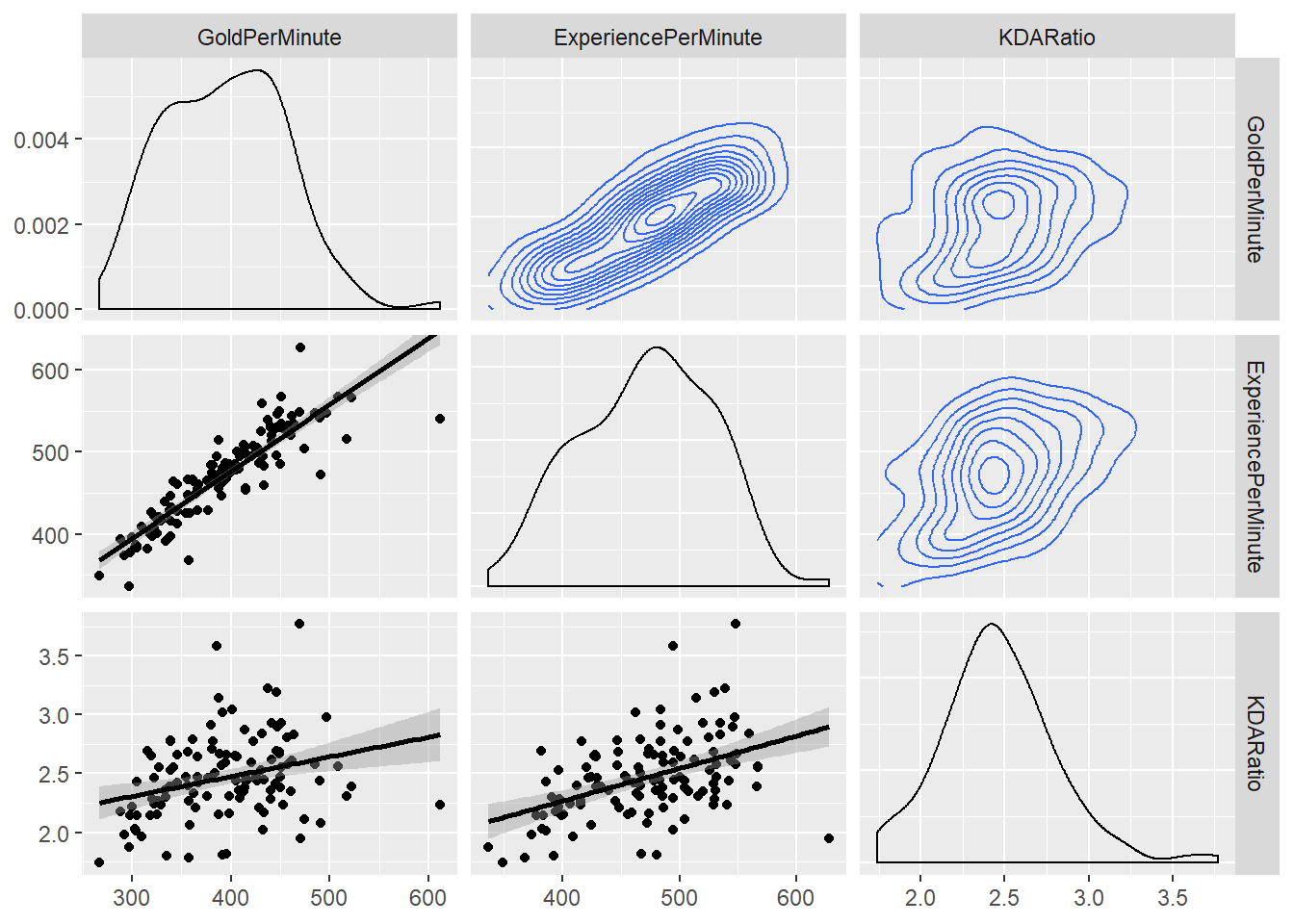### Additional plots - Pairs specific

Packages in R are handy, however, some of them might have limited custamisability. Take ggpairs() as example, we can choose the type of plots for the pairs, this itself is a huge improvement from the base R. However, we can still further improve with this. Some of the possible improvements could be combining the 2D KDE diagram (or contour plot) with a scatterplot - to better visualise the exact position of each of the points, and more importantly, to locate outliers.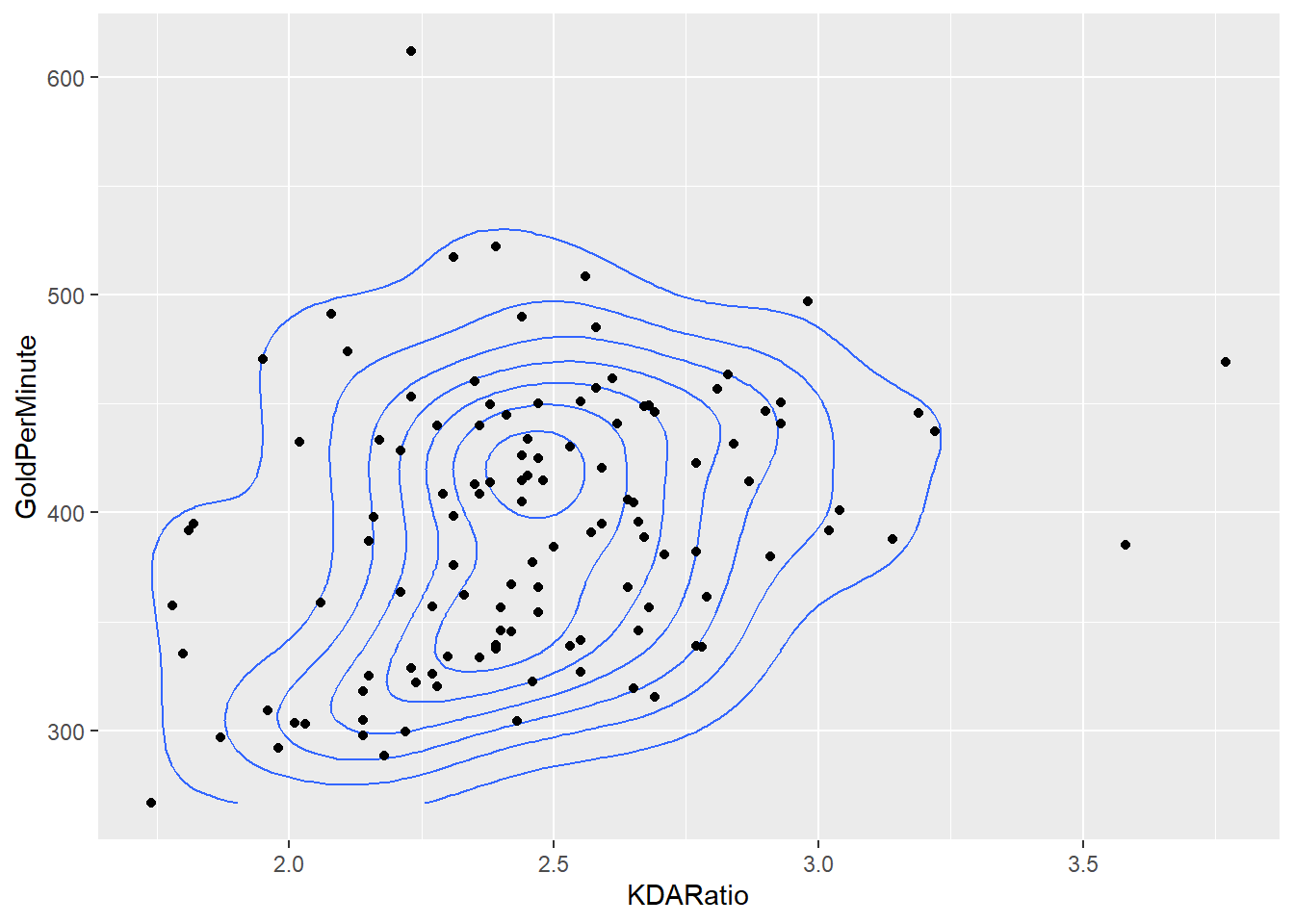Another way to improve the visualisation is the crease a 2D bin diagram (or Heatmap).Each points on the plot represents a bin and the intensity of the color shows the number of actual observation located within the bin.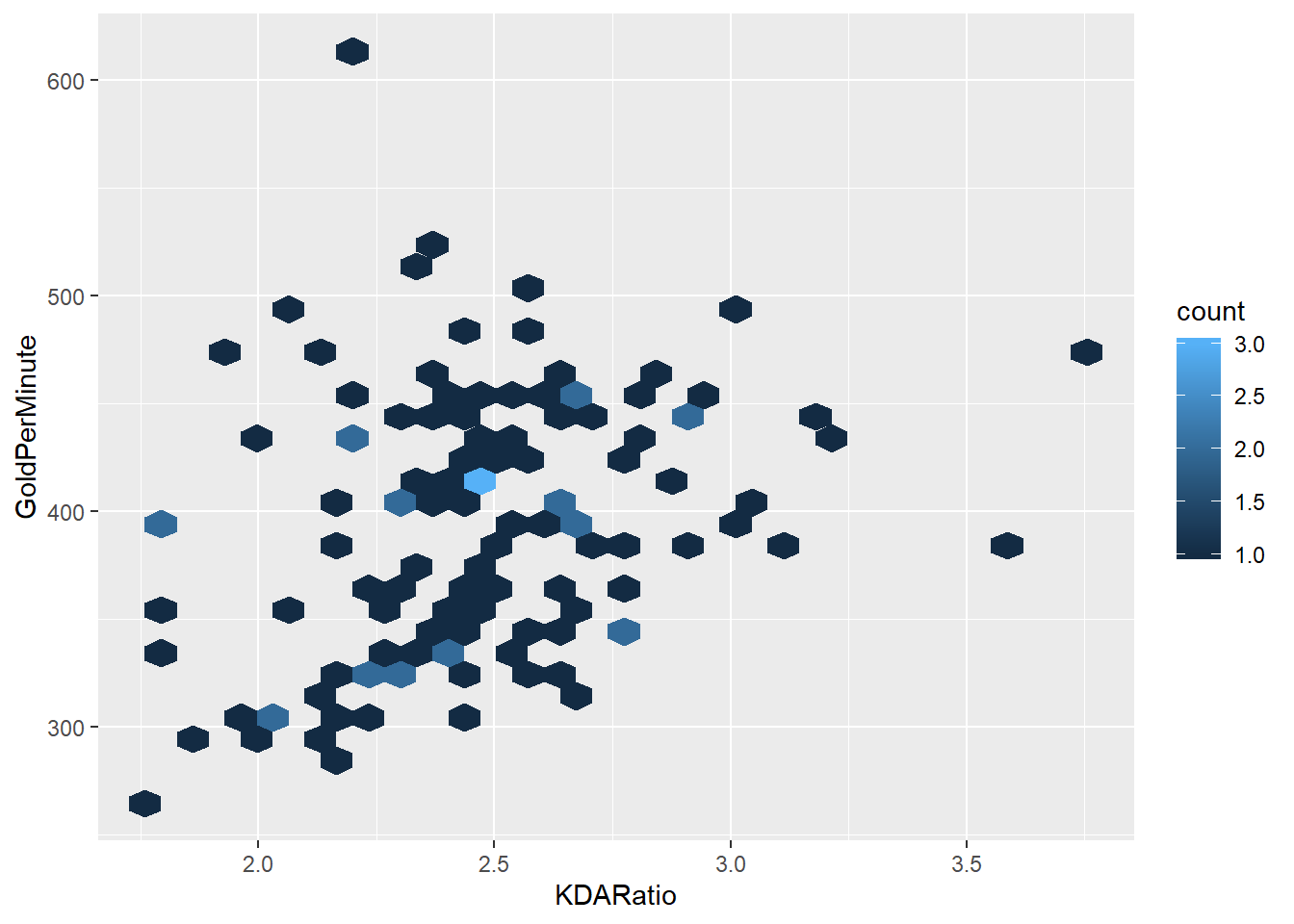More reading on GGally::ggpairs can be found here. https://ggobi.github.io/ggally/#columns_and_mapping